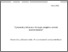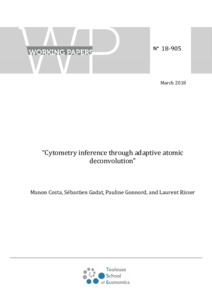# Cytometry inference through adaptive atomic deconvolution

Costa, Manon, Gadat, Sébastien, Gonnord, Pauline and Risser, Laurent (2019) Cytometry inference through adaptive atomic deconvolution. Journal of Nonparametric Statistics, 31 (2). pp. 506-547.Preview
Text

## Abstract

In this paper, we consider a statistical estimation problem known as atomic deconvolution. Introduced in reliability, this model has a direct application when considering biological data produced by flow cytometers. From a statistical point of view, we aim at inferring the percentage of cells expressing the selected molecule and the probability distribution function associated with its fluorescence emission. We propose here an adaptive estimation procedure based on a previous deconvolution procedure introduced by Es, Gugushvili, and Spreij [(2008), â€˜Deconvolution for an atomic distributionâ€™, Electronic Journal of Statistics, 2, 265â€“297] and Gugushvili, Es, and Spreij [(2011), â€˜Deconvolution for an atomic distribution: rates of convergenceâ€™, Journal of Nonparametric Statistics, 23, 1003â€“1029]. For both estimating the mixing parameter and the mixing density automatically, we use the Lepskii method based on the optimal choice of a bandwidth using a bias-variance decomposition. We then derive some convergence rates that are shown to be minimax optimal (up to some log terms) in Sobolev classes. Finally, we apply our algorithm on the simulated and real biological data.

Item Type: Article English April 2019 Yes Mixture models, atomic deconvolution, adaptive kernel estimators, inverse problems B- ECONOMIE ET FINANCE TSE-R (Toulouse) UT1 01 Oct 2019 13:49 01 Oct 2019 13:49 oai:tse-fr.eu:123585 http://publications.ut-capitole.fr/id/eprint/32808

### Available Versions of this ItemView Item